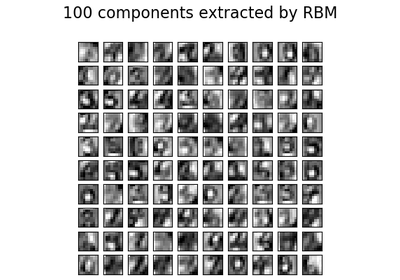# `sklearn.neural_network`.BernoulliRBM¶

class `sklearn.neural_network.``BernoulliRBM`(n_components=256, learning_rate=0.1, batch_size=10, n_iter=10, verbose=0, random_state=None)[source]

Bernoulli Restricted Boltzmann Machine (RBM).

A Restricted Boltzmann Machine with binary visible units and binary hidden units. Parameters are estimated using Stochastic Maximum Likelihood (SML), also known as Persistent Contrastive Divergence (PCD) .

The time complexity of this implementation is `O(d ** 2)` assuming d ~ n_features ~ n_components.

Read more in the User Guide.

Parameters: n_components : int, optional Number of binary hidden units. learning_rate : float, optional The learning rate for weight updates. It is highly recommended to tune this hyper-parameter. Reasonable values are in the 10**[0., -3.] range. batch_size : int, optional Number of examples per minibatch. n_iter : int, optional Number of iterations/sweeps over the training dataset to perform during training. verbose : int, optional The verbosity level. The default, zero, means silent mode. random_state : integer or numpy.RandomState, optional A random number generator instance to define the state of the random permutations generator. If an integer is given, it fixes the seed. Defaults to the global numpy random number generator. intercept_hidden_ : array-like, shape (n_components,) Biases of the hidden units. intercept_visible_ : array-like, shape (n_features,) Biases of the visible units. components_ : array-like, shape (n_components, n_features) Weight matrix, where n_features in the number of visible units and n_components is the number of hidden units.

References

 Hinton, G. E., Osindero, S. and Teh, Y. A fast learning algorithm for
deep belief nets. Neural Computation 18, pp 1527-1554. http://www.cs.toronto.edu/~hinton/absps/fastnc.pdf
 Tieleman, T. Training Restricted Boltzmann Machines using
Approximations to the Likelihood Gradient. International Conference on Machine Learning (ICML) 2008

Examples

```>>> import numpy as np
>>> from sklearn.neural_network import BernoulliRBM
>>> X = np.array([[0, 0, 0], [0, 1, 1], [1, 0, 1], [1, 1, 1]])
>>> model = BernoulliRBM(n_components=2)
>>> model.fit(X)
BernoulliRBM(batch_size=10, learning_rate=0.1, n_components=2, n_iter=10,
random_state=None, verbose=0)
```

Methods

 `fit`(X[, y]) Fit the model to the data X. `fit_transform`(X[, y]) Fit to data, then transform it. `get_params`([deep]) Get parameters for this estimator. `gibbs`(v) Perform one Gibbs sampling step. `partial_fit`(X[, y]) Fit the model to the data X which should contain a partial segment of the data. `score_samples`(X) Compute the pseudo-likelihood of X. `set_params`(**params) Set the parameters of this estimator. `transform`(X) Compute the hidden layer activation probabilities, P(h=1|v=X).
`__init__`(n_components=256, learning_rate=0.1, batch_size=10, n_iter=10, verbose=0, random_state=None)[source]
`fit`(X, y=None)[source]

Fit the model to the data X.

Parameters: X : {array-like, sparse matrix} shape (n_samples, n_features) Training data. self : BernoulliRBM The fitted model.
`fit_transform`(X, y=None, **fit_params)[source]

Fit to data, then transform it.

Fits transformer to X and y with optional parameters fit_params and returns a transformed version of X.

Parameters: X : numpy array of shape [n_samples, n_features] Training set. y : numpy array of shape [n_samples] Target values. X_new : numpy array of shape [n_samples, n_features_new] Transformed array.
`get_params`(deep=True)[source]

Get parameters for this estimator.

Parameters: deep : boolean, optional If True, will return the parameters for this estimator and contained subobjects that are estimators. params : mapping of string to any Parameter names mapped to their values.
`gibbs`(v)[source]

Perform one Gibbs sampling step.

Parameters: v : array-like, shape (n_samples, n_features) Values of the visible layer to start from. v_new : array-like, shape (n_samples, n_features) Values of the visible layer after one Gibbs step.
`partial_fit`(X, y=None)[source]

Fit the model to the data X which should contain a partial segment of the data.

Parameters: X : array-like, shape (n_samples, n_features) Training data. self : BernoulliRBM The fitted model.
`score_samples`(X)[source]

Compute the pseudo-likelihood of X.

Parameters: X : {array-like, sparse matrix} shape (n_samples, n_features) Values of the visible layer. Must be all-boolean (not checked). pseudo_likelihood : array-like, shape (n_samples,) Value of the pseudo-likelihood (proxy for likelihood).

Notes

This method is not deterministic: it computes a quantity called the free energy on X, then on a randomly corrupted version of X, and returns the log of the logistic function of the difference.

`set_params`(**params)[source]

Set the parameters of this estimator.

The method works on simple estimators as well as on nested objects (such as pipelines). The latter have parameters of the form `<component>__<parameter>` so that it’s possible to update each component of a nested object.

Returns: self :
`transform`(X)[source]

Compute the hidden layer activation probabilities, P(h=1|v=X).

Parameters: X : {array-like, sparse matrix} shape (n_samples, n_features) The data to be transformed. h : array, shape (n_samples, n_components) Latent representations of the data.

## Examples using `sklearn.neural_network.BernoulliRBM`¶Restricted Boltzmann Machine features for digit classification